Home Practice
For learners and parents For teachers and schools
Textbooks
Full catalogue
Pricing SupportLog in

We think you are located in United States. Is this correct?

# 5.5 The sine function

## 5.5 The sine function (EMBGW)

### Revision (EMBGX)

#### Functions of the form $$y = \sin \theta$$ for $$\text{0}\text{°} \leq \theta \leq \text{360}\text{°}$$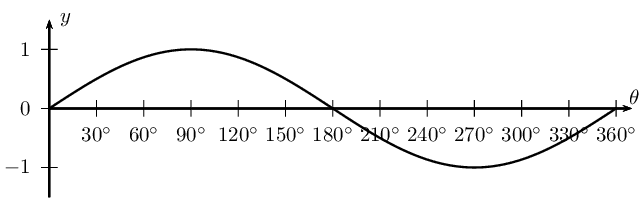• Period of one complete wave is $$\text{360}$$$$\text{°}$$.

• Amplitude is the maximum height of the wave above and below the $$x$$-axis and is always positive. Amplitude = $$\text{1}$$.

• Domain: $$[\text{0}\text{°};\text{360}\text{°}]$$

For $$y = \sin \theta$$, the domain is $$\{ \theta: \theta \in \mathbb{R} \}$$, however in this case, the domain has been restricted to the interval $$\text{0}\text{°} \leq \theta \leq \text{360}\text{°}$$.

• Range: $$\left[-1;1\right]$$

• $$x$$-intercepts: $$\left(\text{0}\text{°};0\right)$$, $$\left(\text{180}\text{°};0\right)$$, $$\left(\text{360}\text{°};0\right)$$

• $$y$$-intercept: $$\left(\text{0}\text{°};0\right)$$

• Maximum turning point: $$\left(\text{90}\text{°};1\right)$$

• Minimum turning point: $$\left(\text{270}\text{°};-1\right)$$

#### Functions of the form $$y = a \sin \theta + q$$

The effects of $$a$$ and $$q$$ on $$f(\theta) = a \sin \theta + q$$:

• The effect of $$q$$ on vertical shift

• For $$q>0$$, $$f(\theta)$$ is shifted vertically upwards by $$q$$ units.

• For $$q<0$$, $$f(\theta)$$ is shifted vertically downwards by $$q$$ units.

• The effect of $$a$$ on shape

• For $$a>1$$, the amplitude of $$f(\theta)$$ increases.

• For $$0<a<1$$, the amplitude of $$f(\theta)$$ decreases.

• For $$a<0$$, there is a reflection about the $$x$$-axis.

• For $$-1 < a < 0$$, there is a reflection about the $$x$$-axis and the amplitude decreases.

• For $$a < -1$$, there is a reflection about the $$x$$-axis and the amplitude increases.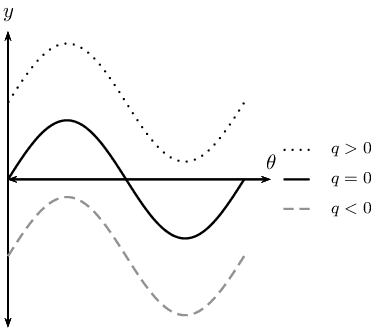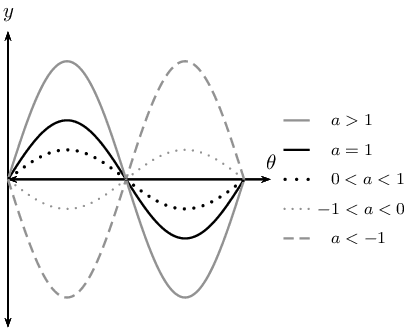temp text

## Revision

Textbook Exercise 5.20

On separate axes, accurately draw each of the following functions for $$\text{0}\text{°} \leq \theta \leq \text{360}\text{°}$$.

• Use tables of values if necessary.
• Use graph paper if available.

For each function also determine the following:

• Period
• Amplitude
• Domain and range
• $$x$$- and $$y$$-intercepts
• Maximum and minimum turning points

$$y_1 = \sin \theta$$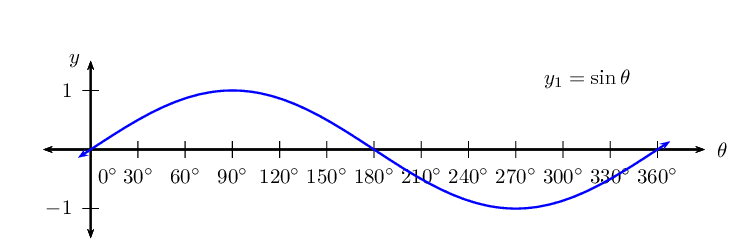$$y_2 = - 2 \sin \theta$$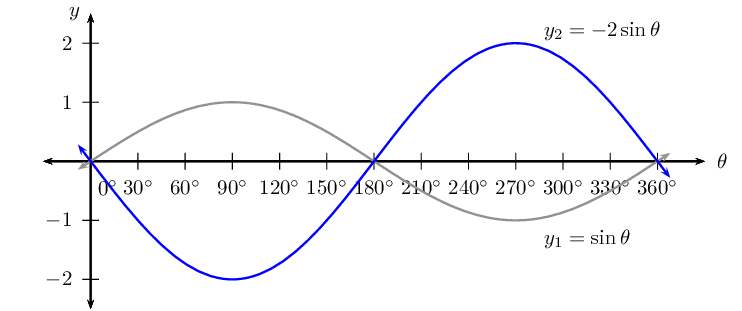$$y_3 = \sin \theta + 1$$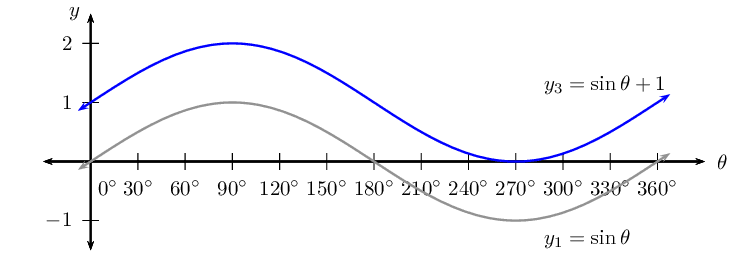$$y_4 = \frac{1}{2} \sin \theta - 1$$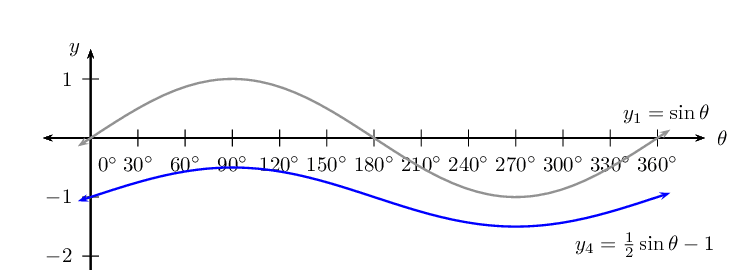## The effects of $$k$$ on a sine graph

1. Complete the following table for $$y_1 = \sin \theta$$ for $$-\text{360}\text{°} \leq \theta \leq \text{360}\text{°}$$:
 $$θ$$ $$-\text{360}$$$$\text{°}$$ $$-\text{270}$$$$\text{°}$$ $$-\text{180}$$$$\text{°}$$ $$-\text{90}$$$$\text{°}$$ $$\text{0}$$$$\text{°}$$ $$\text{90}$$$$\text{°}$$ $$\text{180}$$$$\text{°}$$ $$\text{270}$$$$\text{°}$$ $$\text{360}$$$$\text{°}$$ $$\sin \theta$$
2. Use the table of values to plot the graph of $$y_1 = \sin \theta$$ for $$-\text{360}\text{°} \leq \theta \leq \text{360}\text{°}$$.

3. On the same system of axes, plot the following graphs:

1. $$y_2 = \sin (-\theta)$$
2. $$y_3 = \sin 2\theta$$
3. $$y_4 = \sin \frac{\theta}{2}$$
4. Use your sketches of the functions above to complete the following table:

 $$y_1$$ $$y_2$$ $$y_3$$ $$y_4$$ period amplitude domain range maximum turning points minimum turning points $$y$$-intercept(s) $$x$$-intercept(s) effect of $$k$$
5. What do you notice about $$y_1 = \sin \theta$$ and $$y_2 = \sin (-\theta)$$?

6. Is $$\sin (-\theta) = -\sin \theta$$ a true statement? Explain your answer.

7. Can you deduce a formula for determining the period of $$y = \sin k\theta$$?

The effect of the parameter on $$y = \sin k\theta$$

The value of $$k$$ affects the period of the sine function. If $$k$$ is negative, then the graph is reflected about the $$y$$-axis.

• For $$k > 0$$:

For $$k > 1$$, the period of the sine function decreases.

For $$0 < k < 1$$, the period of the sine function increases.

• For $$k < 0$$:

For $$-1 < k < 0$$, the graph is reflected about the $$y$$-axis and the period increases.

For $$k < -1$$, the graph is reflected about the $$y$$-axis and the period decreases.

Negative angles: $\sin (-\theta) = -\sin \theta$

Calculating the period:

To determine the period of $$y = \sin k\theta$$ we use, $\text{Period } = \frac{\text{360}\text{°}}{|k|}$ where $$|k|$$ is the absolute value of $$k$$ (this means that $$k$$ is always considered to be positive).

 $$0 < k < 1$$ $$-1 < k < 0$$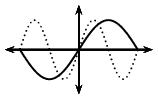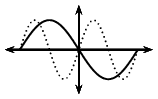$$k > 1$$ $$k < -1$$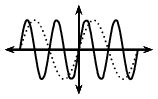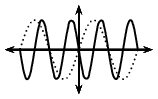## Worked example 18: Sine function

1. Sketch the following functions on the same set of axes for $$-\text{180}\text{°} \leq \theta \leq \text{180}\text{°}$$.
1. $$y_1 = \sin \theta$$
2. $$y_2 = \sin \frac{3\theta}{2}$$
2. For each function determine the following:

1. Period
2. Amplitude
3. Domain and range
4. $$x$$- and $$y$$-intercepts
5. Maximum and minimum turning points

### Examine the equations of the form $$y = \sin k\theta$$

Notice that $$k > 1$$ for $$y_2 = \sin \frac{3\theta}{2}$$, therefore the period of the graph decreases.

### Complete a table of values

 $$θ$$ $$-\text{180}$$$$\text{°}$$ $$-\text{135}$$$$\text{°}$$ $$-\text{90}$$$$\text{°}$$ $$-\text{45}$$$$\text{°}$$ $$\text{0}$$$$\text{°}$$ $$\text{45}$$$$\text{°}$$ $$\text{90}$$$$\text{°}$$ $$\text{135}$$$$\text{°}$$ $$\text{180}$$$$\text{°}$$ $$\sin \theta$$ $$\text{0}$$ $$-\text{0,71}$$ $$-\text{1}$$ $$-\text{0,71}$$ $$\text{0}$$ $$\text{0,71}$$ $$\text{1}$$ $$\text{0,71}$$ $$\text{0}$$ $$\sin \frac{3\theta}{2}$$ $$\text{1}$$ $$\text{0,38}$$ $$-\text{0,71}$$ $$-\text{0,92}$$ $$\text{0}$$ $$\text{0,92}$$ $$\text{0,71}$$ $$-\text{0,38}$$ $$-\text{1}$$

### Sketch the sine graphs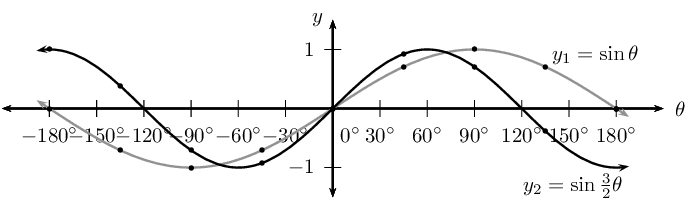### Complete the table

 $$y_1 = \sin \theta$$ $$y_2 = \sin \frac{3\theta}{2}$$ period $$\text{360}$$$$\text{°}$$ $$\text{240}$$$$\text{°}$$ amplitude $$\text{1}$$ $$\text{1}$$ domain $$[-\text{180}\text{°};\text{180}\text{°}]$$ $$[-\text{180}\text{°};\text{180}\text{°}]$$ range $$[-1;1]$$ $$[-1;1]$$ maximum turning points $$(\text{90}\text{°};1)$$ $$(-\text{180}\text{°};1)$$ and $$(\text{60}\text{°};1)$$ minimum turning points $$(-\text{90}\text{°};-1)$$ $$(-\text{60}\text{°};-1) \text{ and } (\text{180}\text{°};1)$$ $$y$$-intercept(s) $$(\text{0}\text{°};0)$$ $$(\text{0}\text{°};0)$$ $$x$$-intercept(s) $$(-\text{180}\text{°};0)$$, $$(\text{0}\text{°};0)$$ and $$(\text{180}\text{°};0)$$ $$(-\text{120}\text{°};0)$$, $$(\text{0}\text{°};0)$$ and $$(\text{120}\text{°};0)$$

#### Discovering the characteristics

For functions of the general form: $$f(\theta) = y =\sin k\theta$$:

Domain and range

The domain is $$\{ \theta: \theta \in \mathbb{R} \}$$ because there is no value for $$\theta$$ for which $$f(\theta)$$ is undefined.

The range is $$\{ f(\theta): -1 \leq f(\theta) \leq 1, f(\theta) \in \mathbb{R} \}$$ or $$[-1;1]$$.

Intercepts

The $$x$$-intercepts are determined by letting $$f(\theta) = 0$$ and solving for $$\theta$$.

The $$y$$-intercept is calculated by letting $$\theta = \text{0}\text{°}$$ and solving for $$f(\theta)$$. \begin{align*} y &= \sin k\theta \\ &= \sin \text{0}\text{°} \\ &= 0 \end{align*} This gives the point $$(\text{0}\text{°};0)$$.

temp text

## Sine functions of the form $$y = \sin k\theta$$

Textbook Exercise 5.21

Sketch the following functions for $$-\text{180}\text{°} \leq \theta \leq \text{180}\text{°}$$ and for each graph determine:

• Period
• Amplitude
• Domain and range
• $$x$$- and $$y$$-intercepts
• Maximum and minimum turning points

$$f(\theta) =\sin 3\theta$$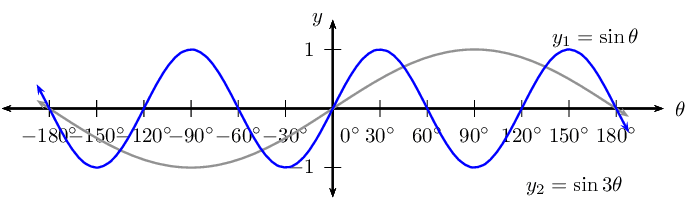For $$f(\theta) =\sin 3\theta$$:

\begin{align*} \text{Period: } & \text{120}\text{°} \\ \text{Amplitude: } & 1 \\ \text{Domain: } & [-\text{180}\text{°};\text{180}\text{°}] \\ \text{Range: } & [-1;1] \\ x\text{-intercepts: } & (-\text{180}\text{°};0); (-\text{120}\text{°};0); (-\text{60}\text{°};0); \\ & (\text{0}\text{°};0); (\text{60}\text{°};0); (\text{120}\text{°};0); (\text{180}\text{°};0) \\ y\text{-intercepts: } & (\text{0}\text{°};0) \\ \text{Max. turning point: } & (-\text{90}\text{°};1); (\text{30}\text{°};1); (\text{150}\text{°};1) \\ \text{Min. turning point: } & (-\text{150}\text{°};-1); (-\text{30}\text{°};-1); (\text{90}\text{°};-1) \end{align*}

$$g(\theta) =\sin \frac{\theta}{3}$$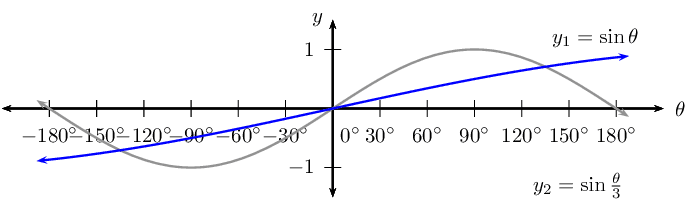For $$g(\theta) =\sin \frac{\theta}{3}$$:

\begin{align*} \text{Period: } & \text{1 080}\text{°} \\ \text{Amplitude: } & 1 \\ \text{Domain: } & [-\text{180}\text{°};\text{180}\text{°}] \\ \text{Range: } & [-\text{0,87};\text{0,87}] \\ x\text{-intercepts: } & \text{ none } \\ y\text{-intercepts: } & (\text{0}\text{°};0) \\ \text{Max. turning point: } & \text{ none } \\ \text{Min. turning point: } & \text{ none } \end{align*}

$$h(\theta) = \sin (-2\theta)$$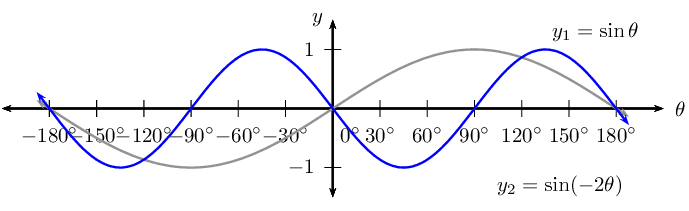For $$h(\theta) =\sin (-2\theta)$$:

\begin{align*} \text{Period: } & \text{180}\text{°} \\ \text{Amplitude: } & 1 \\ \text{Domain: } & [-\text{180}\text{°};\text{180}\text{°}] \\ \text{Range: } & [-1;1] \\ x\text{-intercepts: } & (-\text{180}\text{°};0); (-\text{90}\text{°};0); (\text{0}\text{°};0); (\text{90}\text{°};0); (\text{180}\text{°};0) \\ y\text{-intercepts: } & (\text{0}\text{°};0) \\ \text{Max. turning point: } & (-\text{45}\text{°};1); (\text{135}\text{°};1) \\ \text{Min. turning point: } & (-\text{135}\text{°};-1); (\text{45}\text{°};-1); \end{align*}

$$k(\theta) =\sin \frac{3\theta}{4}$$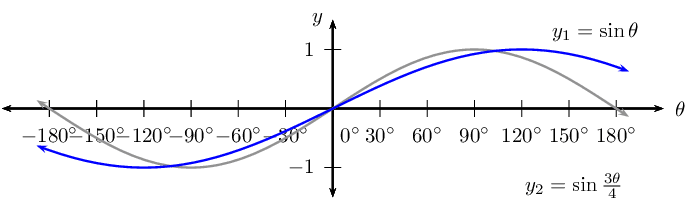For $$k(\theta) =\sin \frac{3\theta}{4}$$:

\begin{align*} \text{Period: } & \text{480}\text{°} \\ \text{Amplitude: } & 1 \\ \text{Domain: } & [-\text{180}\text{°};\text{180}\text{°}] \\ \text{Range: } & [-1;1] \\ x\text{-intercepts: } & (\text{0}\text{°};0) \\ y\text{-intercepts: } & (\text{0}\text{°};0) \\ \text{Max. turning point: } & (\text{120}\text{°};1) \\ \text{Min. turning point: } & (-\text{120}\text{°};-1); \end{align*}

For each graph of the form $$f(\theta) =\sin k\theta$$, determine the value of $$k$$: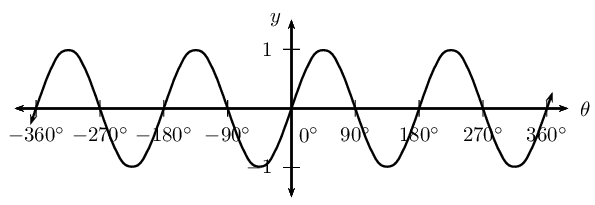\begin{align*} \text{Period } &= \text{180}\text{°} \\ \therefore \frac{\text{360}\text{°}}{k} &= \text{180}\text{°} \\ k &= \frac{\text{360}\text{°}}{\text{180}\text{°}} \\ \therefore k &= 2 \end{align*}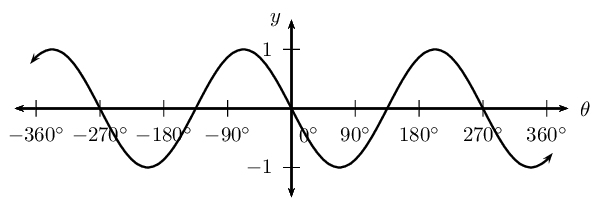\begin{align*} \text{Period } &= \text{270}\text{°} \\ \therefore \frac{\text{360}\text{°}}{k} &= \text{270}\text{°} \\ k &= \frac{\text{360}\text{°}}{\text{270}\text{°}} \\ \therefore k &= \frac{3}{4} \\ \text{and graph is reflected about the } x-\text{axis } \therefore k &= -\frac{3}{4} \end{align*}

## The effects of $$p$$ on a sine graph

1. On the same system of axes, plot the following graphs for $$-\text{360}\text{°} \leq \theta \leq \text{360}\text{°}$$:

1. $$y_1 = \sin \theta$$
2. $$y_2 = \sin (\theta - \text{90}\text{°})$$
3. $$y_3 = \sin (\theta - \text{60}\text{°})$$
4. $$y_4 = \sin (\theta + \text{90}\text{°})$$
5. $$y_5 = \sin (\theta + \text{180}\text{°})$$
2. Use your sketches of the functions above to complete the following table:

 $$y_1$$ $$y_2$$ $$y_3$$ $$y_4$$ $$y_5$$ period amplitude domain range maximum turning points minimum turning points $$y$$-intercept(s) $$x$$-intercept(s) effect of $$p$$

The effect of the parameter on $$y = \sin(\theta + p)$$

The effect of $$p$$ on the sine function is a horizontal shift, also called a phase shift; the entire graph slides to the left or to the right.

• For $$p > 0$$, the graph of the sine function shifts to the left by $$p$$.

• For $$p < 0$$, the graph of the sine function shifts to the right by $$p$$.

 $$p>0$$ $$p<0$$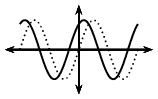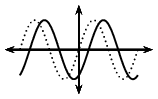## Worked example 19: Sine function

1. Sketch the following functions on the same set of axes for $$-\text{360}\text{°} \leq \theta \leq \text{360}\text{°}$$.
1. $$y_1 = \sin \theta$$
2. $$y_2 = \sin (\theta - \text{30}\text{°})$$
2. For each function determine the following:

1. Period
2. Amplitude
3. Domain and range
4. $$x$$- and $$y$$-intercepts
5. Maximum and minimum turning points

### Examine the equations of the form $$y = \sin (\theta + p)$$

Notice that for $$y_1 = \sin \theta$$ we have $$p = 0$$ (no phase shift) and for $$y_2 = \sin (\theta - \text{30}\text{°})$$, $$p < 0$$ therefore the graph shifts to the right by $$\text{30}\text{°}$$.

### Complete a table of values

 θ $$-\text{360}$$$$\text{°}$$ $$-\text{270}$$$$\text{°}$$ $$-\text{180}$$$$\text{°}$$ $$-\text{90}$$$$\text{°}$$ $$\text{0}$$$$\text{°}$$ $$\text{90}$$$$\text{°}$$ $$\text{180}$$$$\text{°}$$ $$\text{270}$$$$\text{°}$$ $$\text{360}$$$$\text{°}$$ $$\sin \theta$$ $$\text{0}$$ $$\text{1}$$ $$\text{0}$$ $$-\text{1}$$ $$\text{0}$$ $$\text{1}$$ $$\text{0}$$ $$-\text{1}$$ $$\text{0}$$ $$\sin(\theta - \text{30}\text{°})$$ $$-\text{0,5}$$ $$\text{0,87}$$ $$\text{0,5}$$ $$-\text{0,87}$$ $$-\text{0,5}$$ $$\text{0,87}$$ $$\text{0,5}$$ $$-\text{0,87}$$ $$-\text{0,5}$$

### Sketch the sine graphs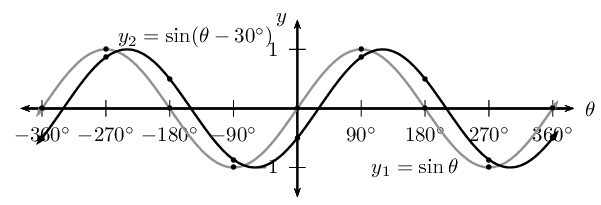### Complete the table

 $$y_1 = \sin \theta$$ $$y_2 = \sin (\theta - \text{30}\text{°})$$ period $$\text{360}\text{°}$$ $$\text{360}\text{°}$$ amplitude $$\text{1}$$ $$\text{1}$$ domain $$[-\text{360}\text{°};\text{360}\text{°}]$$ $$[-\text{360}\text{°};\text{360}\text{°}]$$ range $$[-1;1]$$ $$[-1;1]$$ maximum turning points $$(-\text{270}\text{°};1)$$ and $$(\text{90}\text{°};1)$$ $$(-\text{240}\text{°};1)$$ and $$(\text{120}\text{°};1)$$ minimum turning points $$(-\text{90}\text{°};-1)$$ and $$(\text{270}\text{°};-1)$$ $$(-\text{60}\text{°};-1)$$ and $$(\text{300}\text{°};-1)$$ $$y$$-intercept(s) $$(\text{0}\text{°};0)$$ $$(\text{0}\text{°};-\frac{1}{2})$$ $$x$$-intercept(s) $$(-\text{360}\text{°};0)$$, $$(-\text{180}\text{°};0)$$, $$(\text{0}\text{°};0)$$, $$(\text{180}\text{°};0)$$ and $$(\text{360}\text{°};0)$$ $$(-\text{330}\text{°};0)$$, $$(-\text{150}\text{°};0)$$, $$(\text{30}\text{°};0)$$ and $$(\text{210}\text{°};0)$$

#### Discovering the characteristics

For functions of the general form: $$f(\theta) = y =\sin (\theta + p)$$:

Domain and range

The domain is $$\{ \theta: \theta \in \mathbb{R} \}$$ because there is no value for $$\theta$$ for which $$f(\theta)$$ is undefined.

The range is $$\{ f(\theta): -1 \leq f(\theta) \leq 1, f(\theta) \in \mathbb{R} \}$$.

Intercepts

The $$x$$-intercepts are determined by letting $$f(\theta) = 0$$ and solving for $$\theta$$.

The $$y$$-intercept is calculated by letting $$\theta = \text{0}\text{°}$$ and solving for $$f(\theta)$$.

temp text

## Sine functions of the form $$y = \sin (\theta + p)$$

Textbook Exercise 5.22

Sketch the following functions for $$-\text{360}\text{°} \leq \theta \leq \text{360}\text{°}$$.

For each function, determine the following:

• Period
• Amplitude
• Domain and range
• $$x$$- and $$y$$-intercepts
• Maximum and minimum turning points

$$f(\theta) =\sin (\theta + \text{30}\text{°})$$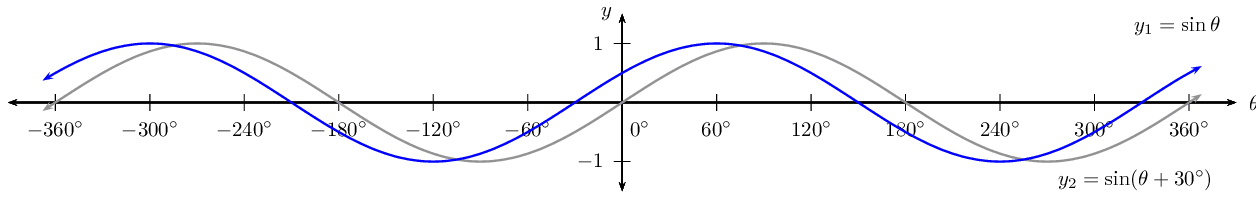For $$f(\theta) =\sin (\theta + \text{30}\text{°})$$:

\begin{align*} \text{Period: } & \text{360}\text{°} \\ \text{Amplitude: } & 1 \\ \text{Domain: } & [-\text{360}\text{°};\text{360}\text{°}] \\ \text{Range: } & [-1;1] \\ x\text{-intercepts: } & (-\text{210}\text{°};0); (-\text{30}\text{°};0); (\text{150}\text{°};0); (\text{330}\text{°};0) \\ y\text{-intercepts: } & (\text{0}\text{°};\frac{1}{2}) \\ \text{Max. turning point: } & (-\text{300}\text{°};1); (\text{60}\text{°};1) \\ \text{Min. turning point: } & (-\text{120}\text{°};-1); (\text{240}\text{°};-1) \end{align*}

$$g(\theta) =\sin (\theta - \text{45}\text{°})$$For $$g(\theta) =\sin (\theta - \text{45}\text{°})$$:

\begin{align*} \text{Period: } & \text{360}\text{°} \\ \text{Amplitude: } & 1 \\ \text{Domain: } & [-\text{360}\text{°};\text{360}\text{°}] \\ \text{Range: } & [-1;1] \\ x\text{-intercepts: } & (-\text{315}\text{°};0); (-\text{135}\text{°};0); (\text{45}\text{°};0); (\text{225}\text{°};0) \\ y\text{-intercepts: } & (\text{0}\text{°};\frac{1}{\sqrt{2}}) \\ \text{Max. turning point: } & (-\text{300}\text{°};1); (-\text{225}\text{°};1) ; (\text{135}\text{°};1) \\ \text{Min. turning point: } & (-\text{45}\text{°};-1); (\text{315}\text{°};-1) \end{align*}

$$h(\theta) =\sin (\theta + \text{60}\text{°})$$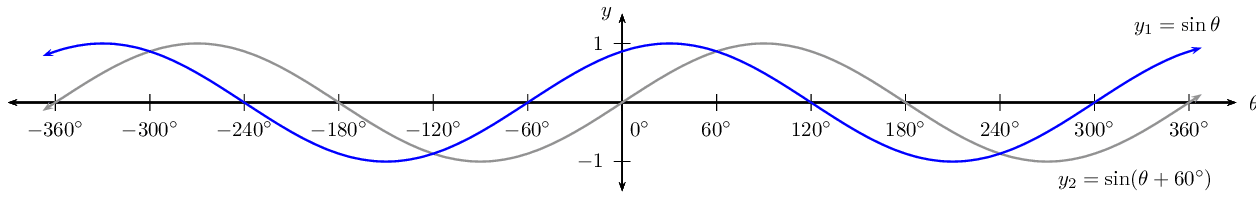For $$h(\theta) =\sin (\theta + \text{60}\text{°})$$:

\begin{align*} \text{Period: } & \text{360}\text{°} \\ \text{Amplitude: } & 1 \\ \text{Domain: } & [-\text{360}\text{°};\text{360}\text{°}] \\ \text{Range: } & [-1;1] \\ x\text{-intercepts: } & (-\text{240}\text{°};0); (-\text{60}\text{°};0); (\text{120}\text{°};0); (\text{300}\text{°};0) \\ y\text{-intercepts: } & (\text{0}\text{°};\frac{\sqrt{3}}{2}) \\ \text{Max. turning point: } & (-\text{300}\text{°};1); (-\text{330}\text{°};1) ; (\text{30}\text{°};1) \\ \text{Min. turning point: } & (-\text{150}\text{°};-1); (\text{210}\text{°};-1) \end{align*}

## Worked example 20: Sketching a sine graph

Sketch the graph of $$f(\theta) = \sin (\text{45}\text{°} - \theta)$$ for $$\text{0}\text{°} \leq \theta \leq \text{360}\text{°}$$.

### Examine the form of the equation

Write the equation in the form $$y = \sin (\theta + p)$$.

\begin{align*} f(\theta) &= \sin (\text{45}\text{°} - \theta)\\ &= \sin (-\theta + \text{45}\text{°}) \\ &= \sin \left( -(\theta - \text{45}\text{°}) \right) \\ &= -\sin (\theta - \text{45}\text{°}) \end{align*}

To draw a graph of the above function, we know that the standard sine graph, $$y = \sin\theta$$, must:

• be reflected about the $$x$$-axis
• be shifted to the right by $$\text{45}\text{°}$$

### Complete a table of values

 θ $$\text{0}$$$$\text{°}$$ $$\text{45}$$$$\text{°}$$ $$\text{90}$$$$\text{°}$$ $$\text{135}$$$$\text{°}$$ $$\text{180}$$$$\text{°}$$ $$\text{225}$$$$\text{°}$$ $$\text{270}$$$$\text{°}$$ $$\text{315}$$$$\text{°}$$ $$\text{360}$$$$\text{°}$$ $$f(\theta)$$ $$\text{0,71}$$ $$\text{0}$$ $$-\text{0,71}$$ $$-\text{1}$$ $$-\text{0,71}$$ $$\text{0}$$ $$\text{0,71}$$ $$\text{1}$$ $$\text{0,71}$$

### Plot the points and join with a smooth curve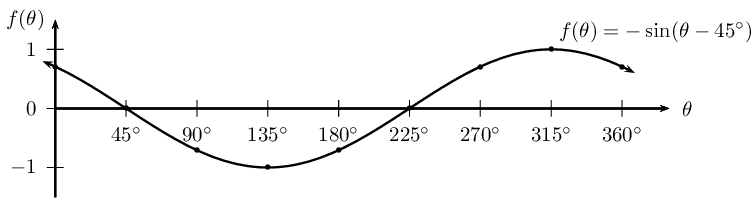Period: $$\text{360}\text{°}$$

Amplitude: $$\text{1}$$

Domain: $$[-\text{360}\text{°};\text{360}\text{°}]$$

Range: $$[-1;1]$$

Maximum turning point: $$(\text{315}\text{°};1)$$

Minimum turning point: $$(\text{135}\text{°};-1)$$

$$y$$-intercepts: $$(\text{0}\text{°};\text{0,71})$$

$$x$$-intercept: $$(\text{45}\text{°};0) \text{ and } (\text{225}\text{°};0)$$

## Worked example 21: Sketching a sine graph

Sketch the graph of $$f(\theta) = \sin (3\theta + \text{60}\text{°})$$ for $$\text{0}\text{°} \leq \theta \leq \text{180}\text{°}$$.

### Examine the form of the equation

Write the equation in the form $$y = \sin k(\theta + p)$$.

\begin{align*} f(\theta) &= \sin (3\theta + \text{60}\text{°})\\ &= \sin 3(\theta + \text{20}\text{°}) \end{align*}

To draw a graph of the above equation, the standard sine graph, $$y = \sin\theta$$, must be changed in the following ways:

• decrease the period by a factor of $$\text{3}$$;
• shift to the left by $$\text{20}\text{°}$$.

### Complete a table of values

 θ $$\text{0}$$$$\text{°}$$ $$\text{30}$$$$\text{°}$$ $$\text{60}$$$$\text{°}$$ $$\text{90}$$$$\text{°}$$ $$\text{120}$$$$\text{°}$$ $$\text{150}$$$$\text{°}$$ $$\text{180}$$$$\text{°}$$ $$f(\theta)$$ $$\text{0,87}$$ $$\text{0,5}$$ $$-\text{0,87}$$ $$-\text{0,5}$$ $$\text{0,87}$$ $$\text{0,5}$$ $$-\text{0,87}$$

### Plot the points and join with a smooth curve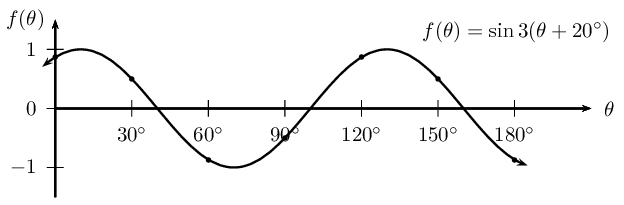Period: $$\text{120}\text{°}$$

Amplitude: $$\text{1}$$

Domain: $$[\text{0}\text{°}; \text{180}\text{°}]$$

Range: $$[-1;1]$$

Maximum turning point: $$(\text{10}\text{°}; 1) \text{ and } (\text{130}\text{°}; 1)$$

Minimum turning point: $$(\text{70}\text{°}; -1)$$

$$y$$-intercept: $$(\text{0}\text{°}; \text{0,87})$$

$$x$$-intercepts: $$(\text{40}\text{°}; 0)$$, $$(\text{100}\text{°}; 0)$$ and $$(\text{160}\text{°}; 0)$$

## The sine function

Textbook Exercise 5.23

Sketch the following graphs on separate axes:

$$y = 2 \sin \frac{\theta}{2}$$ for $$-\text{360}\text{°} \leq \theta \leq \text{360}\text{°}$$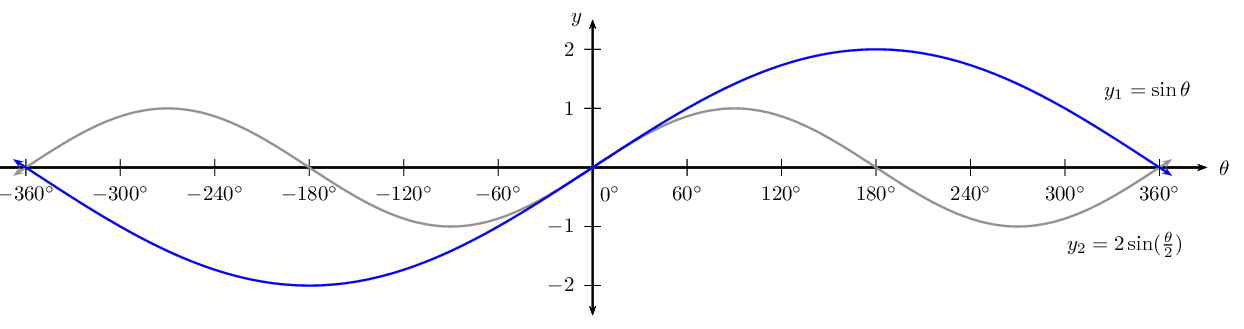$$f(\theta) = \frac{1}{2} \sin (\theta - \text{45}\text{°})$$ for $$-\text{90}\text{°} \leq \theta \leq \text{90}\text{°}$$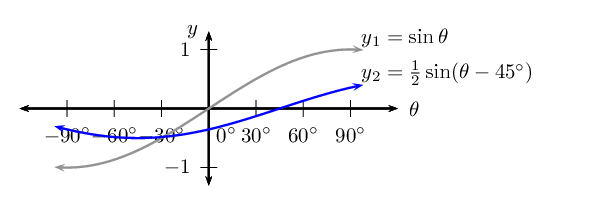$$y = \sin (\theta + \text{90}\text{°}) + 1$$ for $$\text{0}\text{°} \leq \theta \leq \text{360}\text{°}$$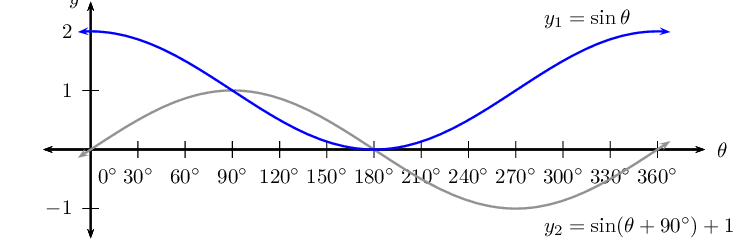$$y = \sin (-\frac{3\theta}{2})$$ for $$-\text{180}\text{°} \leq \theta \leq \text{180}\text{°}$$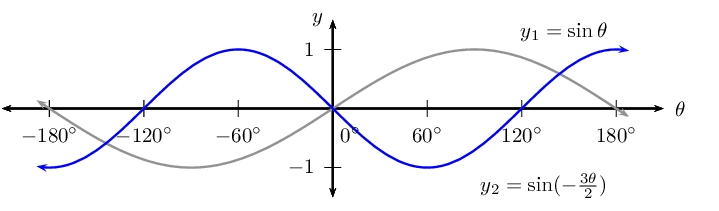$$y = \sin (\text{30}\text{°} - \theta)$$ for $$-\text{360}\text{°} \leq \theta \leq \text{360}\text{°}$$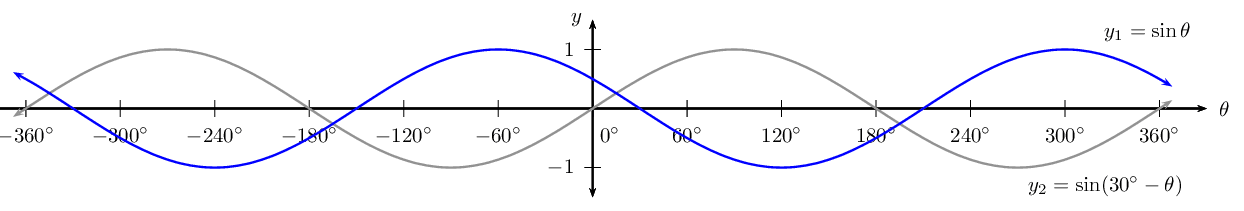Given the graph of the function $$y = a \sin (\theta + p)$$, determine the values of $$a$$ and $$p$$.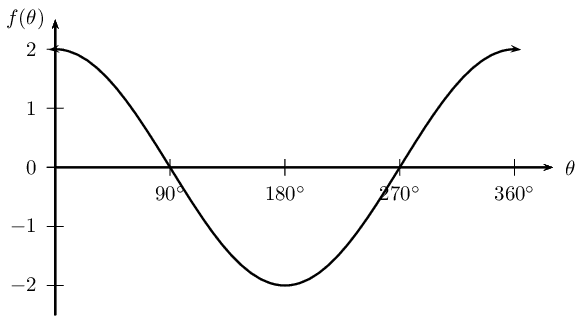Can you describe this graph in terms of $$\cos \theta$$?

$$a = 2$$; $$p = \text{90}\text{°} \therefore y = 2 \sin ( \theta + \text{90}\text{°})$$ and $$y = 2 \cos \theta$$